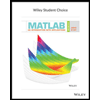# 5.Fowle Marketing Research, Inc., bases charges to a client on the assumption that telephone surveys can be completed within 15 minutes or less. If more time is required, a premium rate is charged. With a sample of 35 surveys, a population standard deviation of 4 minutes, and a level of significance of .01, the sample mean will be used to test the null hypothesis H_0: μ ≤15. What is your interpretation of the Type II error for this problem? What is its impact on the firm? What is the probability of making a Type II error when the actual mean time is μ=17 minutes? What is the probability of making a Type II error when the actual mean time is μ= 18 minutes?

Question
5.Fowle Marketing Research, Inc., bases charges to a client on the assumption that telephone surveys can be completed within 15 minutes or less. If more time is required, a premium rate is charged. With a sample of 35 surveys, a population standard deviation of 4 minutes, and a level of significance of .01, the sample mean will be used to test the null hypothesis H_0: μ ≤15. What is your interpretation of the Type II error for this problem? What is its impact on the firm? What is the probability of making a Type II error when the actual mean time is μ=17 minutes? What is the probability of making a Type II error when the actual mean time is μ= 18 minutes?
Expert Solution

### Want to see the full answer?

Check out a sample Q&A hereStudents who’ve seen this question also like:MATLAB: An Introduction with Applications
6th Edition
ISBN: 9781119256830
Author: Amos Gilat
Publisher: John Wiley & Sons Inc